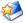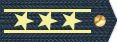# [原创]纵论俄罗斯载人登月火箭的研制技术路径

打赏
收藏文本
1
0
2018/11/28 9:00:54

## 网友回复

•• 军号：1734531
• 工分:101221
左箭头-小图标

经济基础决定上层建筑

俄罗斯这些年放的卫星不少，前些年下水多少艘航母，多少核潜艇，买多少飞机，但是现在呢，啥也没做成

原因很简单：没钱

没钱就别想耍帅，航天是烧钱的大户，没钱是万万不能滴

2019/1/3 9:04:15
左箭头-小图标

[size=10.5]第二节，以俄罗斯的国家体量要长久养活中间运力型火箭非常艰难。[size=10.5]
[size=10.5]
[size=10.5]以俄罗斯东方发射场或者拜科努尔发射场所处的纬度来分析，不使用氢氧上面级，俄罗斯人要堆出[size=10.5]LTO[size=10.5]运力达[size=10.5]15[size=10.5]吨级的中间运力型火箭，其起飞质量也要[size=10.5]2000[size=10.5]至[size=10.5]2500[size=10.5]吨左右了。这已经要比目前版本质子火箭的重量大上[size=10.5]3[size=10.5]倍了，因此那怕是这种级别的火箭俄罗斯政府要长久养活也不是那么轻松的事情。如果未来俄罗斯参与[size=10.5]NRHO[size=10.5]深空站的建设与运营，每年提供[size=10.5]4[size=10.5]枚这种级别的中间运力型火箭的发射量，按目前质子火箭[size=10.5]1[size=10.5]亿美元一枚的价格来分析，每枚[size=10.5]LTO[size=10.5]运力达[size=10.5]15[size=10.5]吨级的火箭的市场价格什么也要[size=10.5]3[size=10.5]亿美元以上，[size=10.5]4[size=10.5]枚火箭就要价高达[size=10.5]12[size=10.5]亿美元了。再加[size=10.5]2[size=10.5]艘联盟载人飞船（月球版）与[size=10.5]2[size=10.5]艘进步飞船（月球版），合计为[size=10.5]8[size=10.5]亿美元。

[size=10.5]如此未来一年俄罗斯政府在[size=10.5]NRHO[size=10.5]与载人登月工程上就起码要花费上[size=10.5]20[size=10.5]亿美元的资金了，这对穷困的俄罗斯政府而言绝对不是一项轻松的负担。[size=10.5]

[size=10.5]
[size=10.5]这个道理放置于欧洲与日本政府的身上也一样成立（但对中国美国政府这一点经济压力并不算大，也就是一艘[size=10.5]055[size=10.5]与伯克[size=10.5]3[size=10.5]驱逐舰的价钱而已）。所以对于俄、日、欧这三者而言，那怕仅仅参与[size=10.5]NRHO[size=10.5]深空站也远远没有想象中的那么轻松与简单，也都是要脱层皮的“大事”。[size=10.5]

[size=10.5]粗略计算下，那怕是通过[size=10.5]NRHO[size=10.5]深空站中转进行载人登月，以每年[size=10.5]2[size=10.5]次月球登陆任务，平均欧、日、俄每国仅[size=10.5]1[size=10.5]名宇航员登陆月球（美国[size=10.5]3[size=10.5]名宇航员）。欧、日、俄每年都至少要花费上[size=10.5]10[size=10.5]亿美元的政府投资预算。而美国则至少要多花[size=10.5]20[size=10.5]亿美元以上。一句话，月球不是那么好登陆的。[size=10.5]
[size=10.5]
[size=10.5]细化分析后，政府主导的载人登月任务基本要[size=10.5]10[size=10.5]亿美元一条票，这要比今天国际空间站的[size=10.5]1[size=10.5]亿美元一条票的价格要贵上[size=10.5]10[size=10.5]倍左右了。[size=10.5]
[size=10.5]
[size=10.5]一款安加拉火箭就让俄罗斯人搞了二十多年才研制成功，俄罗斯国力的持续衰落局面由此可见一斑。如果俄罗斯人再不能够沉下心来踏踏实实研制一款[size=10.5]LTO[size=10.5]运力只有[size=10.5]15[size=10.5]吨的火箭，还是三心两意、虚度时光，那么这一轮载人登月浪潮将注定没有俄罗斯人的什么事了。[size=10.5]
[size=10.5]
[size=10.5]其实那个起飞级一共使用[size=10.5]5[size=10.5]个[size=10.5]CBC[size=10.5]推进模块的联盟[size=10.5]5[size=10.5]火箭才是真正符合俄罗斯人国情的载人登月火箭。在当前资金紧张的环境下，先研制全煤油版本的联盟[size=10.5]5[size=10.5]火箭，争取能够实现将联盟载人飞船的月球版送入[size=10.5]NRHO[size=10.5]轨道，能够在未来[size=10.5]10[size=10.5]年内紧紧跟上美国载人重返月球的步伐再说，这也算优先解决了“有无问题”。更重要的是，起飞级使用[size=10.5]3[size=10.5]个[size=10.5]CBC[size=10.5]模块的联盟[size=10.5]5[size=10.5]火箭的[size=10.5]GTO[size=10.5]运力也有[size=10.5]15[size=10.5]吨以上，这在未来通讯卫星大型化的时代中也能够获得大量的使用机会，从而大大降低这一款火箭的持续生存运营压力。[size=10.5]
[size=10.5]
[size=10.5]至于要研制氢氧上面级来进一步提升这款火箭的[size=10.5]LTO[size=10.5]运力水平到[size=10.5]20[size=10.5]至[size=10.5]25[size=10.5]吨的区间，推迟到[size=10.5]2040[size=10.5]年之后再进行就可以了。[size=10.5]
[size=10.5]
[size=10.5]如果未来（[size=10.5]2050[size=10.5]年后）俄罗斯人真的发了横财之后，也可以研制直径达[size=10.5]6[size=10.5]米的全新第二级（并联使用[size=10.5]4[size=10.5]台[size=10.5]RD191[size=10.5]发动机的高空版），再研制直径同样达[size=10.5]6[size=10.5]米的氢氧第三级（并联使用[size=10.5]5[size=10.5]台[size=10.5]RD0146[size=10.5]氢氧发动机），如此改进后其[size=10.5]LTO[size=10.5]运力要提升到[size=10.5]35[size=10.5]吨区间也不是什么的难事。[size=10.5]
[size=10.5]
[size=10.5]反正联盟[size=10.5]5[size=10.5]火箭起飞级使用的[size=10.5]5[size=10.5]台[size=10.5]RD170[size=10.5]发动机的合计推力己经接近[size=10.5]4000[size=10.5]吨，未来改进的潜力大得很。而且联盟[size=10.5]5[size=10.5]火箭的气动布置也比较接近安加拉[size=10.5]5[size=10.5]火箭，研制的技术风险也非常低。至于捆绑[size=10.5]5[size=10.5]个、[size=10.5]6[size=10.5]个[size=10.5]RD170[size=10.5]煤油发动机[size=10.5]CBC[size=10.5]推进模块的设想，由于气动布置非常复杂，技术方案过于激进，其实都是异端了，搞不好[size=10.5]N1[size=10.5]火箭在地狱里也还会向俄罗斯人的新款载人登月火箭招手。而且起飞级使用[size=10.5]5[size=10.5]个[size=10.5]CBC[size=10.5]模块的联盟[size=10.5]5[size=10.5]火箭，末来也有潜力升级为可重复使用火箭（即[size=10.5]5[size=10.5]个[size=10.5]CBC[size=10.5]模块都可以组合成一个整体，方便一齐回收复用）。[size=10.5]

[size=10.5]陈天（高凉陈君）[size=10.5]
[size=10.5]
[size=10.5]2018[size=10.5]年[size=10.5]11[size=10.5]月[size=10.5]27[size=10.5]日[size=10.5]
[size=10.5]

2018/11/28 9:02:32

总页数11页 [共有3条记录] 分页：

1
对[原创]纵论俄罗斯载人登月火箭的研制技术路径回复